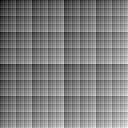# Counting Ones

``````f x y = (* adjust) \$ count (toBits x) + count (toBits y)

adjust = (2 ^ scale) `div` (2 * scale)

count []         = 0
count (True :xs) = 1 + count xs
count (False:xs) =     count xs``````